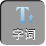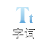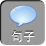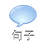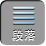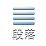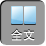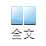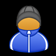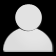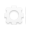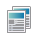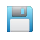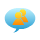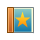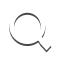-AA+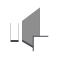1）求回路中感应电动势及感应电流随时间变化的表达式；

2）经过多长时间拉力 F达到最大值，拉力 F的最大值为多少？

3）某过程中回路产生的焦耳热为 Q，导轨克服摩擦力做功为 W，求导轨动能的增加量。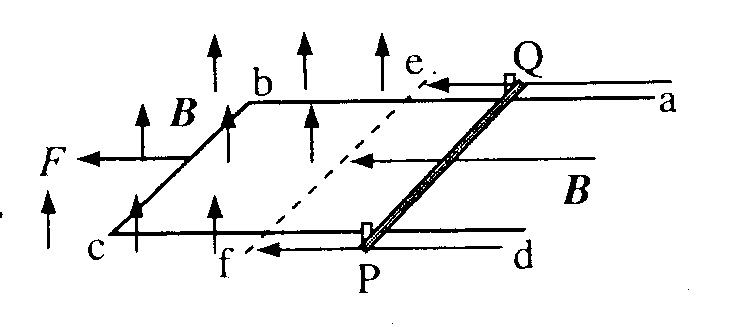1）感应电动势ε= BLv

S= at 2 /2

I= BLv/ R= BLat/[ R+ 2 R0( at 2 /2)]= BLat/( R+ R0 at 2)

2）导轨受外力 F，安培力 F A，摩擦力 F f其中

F A= BIL= B 2 L 2 at/( R+ R0 at 2)

F fF N=μ( mg+ BIL)=μ[ mg+ B 2 L 2 at/( R+ R0 at 2)]

F= Ma+ F A+ F f将以上式子代入可得

R/ t= R0 atF取极大值，此时 t=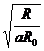3）设在此过程中导轨运动距离 s，由动能定理 WE k

W= Mas

WmgsW AmgsQ

Δ E k= Mas= Ma( W -μ Q)/μ mg